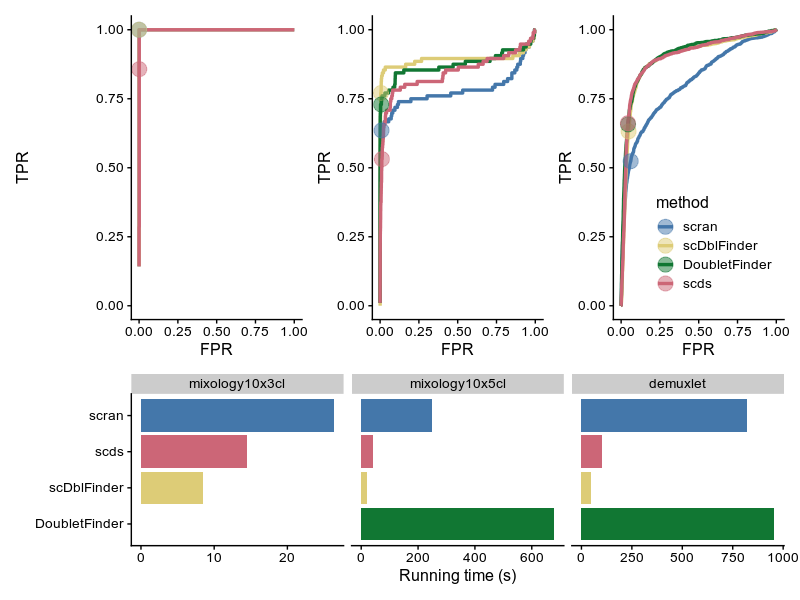# 1 scDblFinder

## 1.1 Introduction

scDblFinder identifies doublets in single-cell RNAseq directly by creating artificial doublets and looking at their prevalence in the neighborhood of each cell. The rough logic is very similar to DoubletFinder, but it is simpler and more efficient. In a nutshell, instead of creating doublets from random pairs of cells, scDblFinder first overclusters the cells and create cross-cluster doublets. It also uses meta-cells from each cluster to create triplets. This strategy avoids creating homotypic doublets and enables the detection of most heterotypic doublets with much fewer artificial doublets. We also rely on the expected proportion of doublets to threshold the scores, we include a variability in the estimate of the doublet proportion (dbr.sd), and use the error rate of the real/artificial predicition in conjunction with the deviation in global doublet rate to set the threshold.

The approach described here is complementary to doublets identified via cell hashes and SNPs in multiplexed samples.
The latter can identify doublets formed by cells of the same type from two samples, which are nearly undistinguishable from real cells transcriptionally (and hence unidentifiable through the present package), but cannot identify doublets made by cells of the same sample.

## 1.2 Installation

scDblFinder was developed under R 3.6. Install with:

if (!requireNamespace("BiocManager", quietly = TRUE))
install.packages("BiocManager")
BiocManager::install("scDblFinder")

# or, to get that latest developments:
BiocManager::install("plger/scDblFinder")

## 1.3 Usage

Given an object sce of class SingleCellExperiment (which does not contain any empty drops, but hasn’t been further filtered) :

# we create a dummy dataset
sce <- scater::mockSCE(ncells=500, ngenes=500)
library(scDblFinder)
sce <- scDblFinder(sce, verbose=FALSE)

This will add the following columns to the colData of sce:

• sce$scDblFinder.ratio : the proportion of artificial doublets among the neighborhood (the higher, the more chances that the cell is a doublet) • sce$scDblFinder.weighted : the proportion of artificial doublets among the neighborhood, weighted by distance
• sce$scDblFinder.score : the final doublet score • sce$scDblFinder.class : the classification (doublet or singlet)

### 1.3.1 Multiple samples

If you have multiple samples (understood as different cell captures), then it is preferable to look for doublets separately for each sample (for multiplexed samples with cell hashes, this means for each batch). You can do this by simply providing a vector of the sample ids to the samples parameter of scDblFinder or, if these are stored in a column of colData, the name of the column. In this case, you might also consider multithreading it using the BPPARAM parameter. For example:

library(BiocParallel)
sce <- scDblFinder(sce, samples="sample_id", BPPARAM=MulticoreParam(3))
table(sce\$scDblFinder.class)

### 1.3.2 Parameters

The important sets of parameters in scDblFinder refer respectively to the expected proportion of doublets, to the clustering, and to the number of artificial doublets used.

#### 1.3.2.1 Expected proportion of doublets

The expected proportion of doublets has no impact on the score (the ratio above), but a very strong impact on where the threshold will be placed. It is specified through the dbr parameter and the dbr.sd parameter (the latter specifies the standard deviation of dbr, i.e. the uncertainty in the expected doublet rate). For 10x data, the more cells you capture the higher the chance of creating a doublet, and Chromium documentation indicates a doublet rate of roughly 1% per 1000 cells captures (so with 5000 cells, (0.01*5)*5000 = 250 doublets), and the default expected doublet rate will be set to this value (with a default standard deviation of 0.015). Note however that different protocols may create considerably more doublets, and that this should be updated accordingly.

#### 1.3.2.2 Clustering

Since doublets are created across clusters, it is important that subpopulations are not misrepresented as belonging to the same cluster. For this reason, we favor over-clustering at this stage. This is for instance implemented by scDblFinder’s overcluster function, and controlled by specifying minimum and maximum cluster sizes. Alternatively, cluster labels can be directly provided.

#### 1.3.2.3 Number of artificial doublets

scDblFinder itself determines a reasonable number of artificial doublets to create on the basis of the size of the population and the number of clusters, but increasing this number can only increase the accuracy.

## 1.4 Combination with other tools

If the input SCE already contains a logcounts and/or reducedDim slot named ‘PCA’, scDblFinder will used them for the clustering step. In addition, a clustering can be manually given using the clusters argument of scDblFinder(). In this way, seurat clustering could for instance be used (in which case we suggest to increase the resolution parameter) to create the artifical doublets (see ?Seurat::as.SingleCellExperiment.Seurat for conversion to SCE).

# 2 Comparison with other doublet callers

To benchmark scDblFinder against alternatives, we used datasets in which cells from multiple individuals were mixed and their identity deconvoluted using SNPs (via demuxlet), which also enables the identification of doublets from different individuals.

The method is compared to:Figure 1: Comparison with other tools; note that doubletFinder failed on the mixology10x3cl dataset